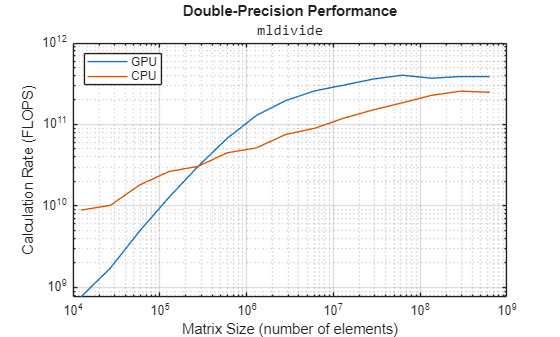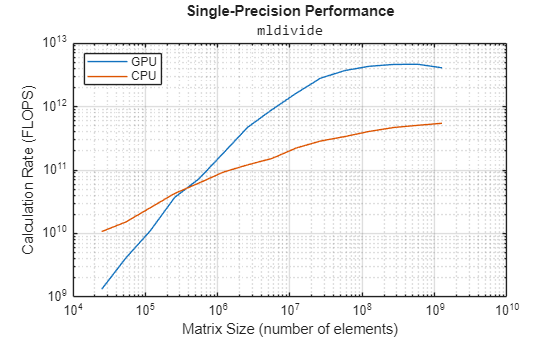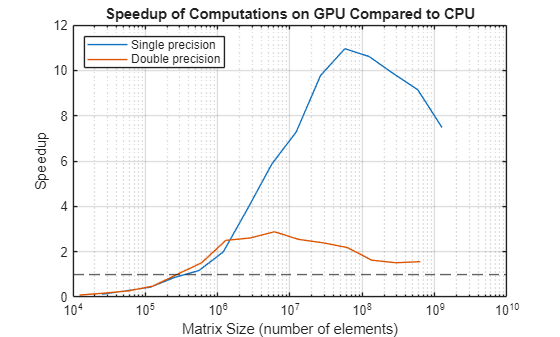# Benchmarking A\b on the GPU

This example looks at how we can benchmark the solving of a linear system on the GPU. The MATLAB® code to solve for `x` in `A*x = b` is very simple. Most frequently, we use matrix left division, also known as `mldivide` or the backslash operator (\), to calculate `x` (that is, `x = A\b`).

Related examples:

The code shown in this example can be found in this function:

```function results = paralleldemo_gpu_backslash(maxMemory) ```

It is important to choose the appropriate matrix size for the computations. We can do this by specifying the amount of system memory in GB available to the CPU and the GPU. The default value is based only on the amount of memory available on the GPU, and you can specify a value that is appropriate for your system.

```if nargin == 0 g = gpuDevice; maxMemory = 0.4*g.AvailableMemory/1024^3; end ```

### The Benchmarking Function

We want to benchmark matrix left division (\), and not the cost of transferring data between the CPU and GPU, the time it takes to create a matrix, or other parameters. We therefore separate the data generation from the solving of the linear system, and measure only the time it takes to do the latter.

```function [A, b] = getData(n, clz) fprintf('Creating a matrix of size %d-by-%d.\n', n, n); A = rand(n, n, clz) + 100*eye(n, n, clz); b = rand(n, 1, clz); end function time = timeSolve(A, b, waitFcn) tic; x = A\b; %#ok<NASGU> We don't need the value of x. waitFcn(); % Wait for operation to complete. time = toc; end ```

### Choosing Problem Size

As with a great number of other parallel algorithms, the performance of solving a linear system in parallel depends greatly on the matrix size. As seen in other examples, such as Benchmarking A\b, we compare the performance of the algorithm for different matrix sizes.

```% Declare the matrix sizes to be a multiple of 1024. maxSizeSingle = floor(sqrt(maxMemory*1024^3/4)); maxSizeDouble = floor(sqrt(maxMemory*1024^3/8)); step = 1024; if maxSizeDouble/step >= 10 step = step*floor(maxSizeDouble/(5*step)); end sizeSingle = 1024:step:maxSizeSingle; sizeDouble = 1024:step:maxSizeDouble; ```

### Comparing Performance: Gigaflops

We use the number of floating point operations per second as our measure of performance because that allows us to compare the performance of the algorithm for different matrix sizes.

Given a matrix size, the benchmarking function creates the matrix `A` and the right-hand side `b` once, and then solves `A\b` a few times to get an accurate measure of the time it takes. We use the floating point operations count of the HPC Challenge, so that for an n-by-n matrix, we count the floating point operations as `2/3*n^3 + 3/2*n^2`.

The function is passed in a handle to a 'wait' function. On the CPU, this function does nothing. On the GPU, this function waits for all pending operations to complete. Waiting in this way ensures accurate timing.

```function gflops = benchFcn(A, b, waitFcn) numReps = 3; time = inf; % We solve the linear system a few times and calculate the Gigaflops % based on the best time. for itr = 1:numReps tcurr = timeSolve(A, b, waitFcn); time = min(tcurr, time); end % Measure the overhead introduced by calling the wait function. tover = inf; for itr = 1:numReps tic; waitFcn(); tcurr = toc; tover = min(tcurr, tover); end % Remove the overhead from the measured time. Don't allow the time to % become negative. time = max(time - tover, 0); n = size(A, 1); flop = 2/3*n^3 + 3/2*n^2; gflops = flop/time/1e9; end % The CPU doesn't need to wait: this function handle is a placeholder. function waitForCpu() end % On the GPU, to ensure accurate timing, we need to wait for the device % to finish all pending operations. function waitForGpu(theDevice) wait(theDevice); end ```

### Executing the Benchmarks

Having done all the setup, it is straightforward to execute the benchmarks. However, the computations can take a long time to complete, so we print some intermediate status information as we complete the benchmarking for each matrix size. We also encapsulate the loop over all the matrix sizes in a function, to benchmark both single- and double-precision computations.

```function [gflopsCPU, gflopsGPU] = executeBenchmarks(clz, sizes) fprintf(['Starting benchmarks with %d different %s-precision ' ... 'matrices of sizes\nranging from %d-by-%d to %d-by-%d.\n'], ... length(sizes), clz, sizes(1), sizes(1), sizes(end), ... sizes(end)); gflopsGPU = zeros(size(sizes)); gflopsCPU = zeros(size(sizes)); gd = gpuDevice; for i = 1:length(sizes) n = sizes(i); [A, b] = getData(n, clz); gflopsCPU(i) = benchFcn(A, b, @waitForCpu); fprintf('Gigaflops on CPU: %f\n', gflopsCPU(i)); A = gpuArray(A); b = gpuArray(b); gflopsGPU(i) = benchFcn(A, b, @() waitForGpu(gd)); fprintf('Gigaflops on GPU: %f\n', gflopsGPU(i)); end end ```

We then execute the benchmarks in single and double precision.

```[cpu, gpu] = executeBenchmarks('single', sizeSingle); results.sizeSingle = sizeSingle; results.gflopsSingleCPU = cpu; results.gflopsSingleGPU = gpu; [cpu, gpu] = executeBenchmarks('double', sizeDouble); results.sizeDouble = sizeDouble; results.gflopsDoubleCPU = cpu; results.gflopsDoubleGPU = gpu; ```
```Starting benchmarks with 7 different single-precision matrices of sizes ranging from 1024-by-1024 to 19456-by-19456. Creating a matrix of size 1024-by-1024. Gigaflops on CPU: 43.805496 Gigaflops on GPU: 78.474002 Creating a matrix of size 4096-by-4096. Gigaflops on CPU: 96.459635 Gigaflops on GPU: 573.278854 Creating a matrix of size 7168-by-7168. Gigaflops on CPU: 184.997657 Gigaflops on GPU: 862.755636 Creating a matrix of size 10240-by-10240. Gigaflops on CPU: 204.404384 Gigaflops on GPU: 978.362901 Creating a matrix of size 13312-by-13312. Gigaflops on CPU: 218.773070 Gigaflops on GPU: 1107.983667 Creating a matrix of size 16384-by-16384. Gigaflops on CPU: 233.529176 Gigaflops on GPU: 1186.423754 Creating a matrix of size 19456-by-19456. Gigaflops on CPU: 241.482550 Gigaflops on GPU: 1199.151846 Starting benchmarks with 5 different double-precision matrices of sizes ranging from 1024-by-1024 to 13312-by-13312. Creating a matrix of size 1024-by-1024. Gigaflops on CPU: 34.902918 Gigaflops on GPU: 72.191488 Creating a matrix of size 4096-by-4096. Gigaflops on CPU: 74.458136 Gigaflops on GPU: 365.339897 Creating a matrix of size 7168-by-7168. Gigaflops on CPU: 93.313782 Gigaflops on GPU: 522.514165 Creating a matrix of size 10240-by-10240. Gigaflops on CPU: 104.219804 Gigaflops on GPU: 628.301313 Creating a matrix of size 13312-by-13312. Gigaflops on CPU: 108.826886 Gigaflops on GPU: 681.881032 ```

### Plotting the Performance

We can now plot the results, and compare the performance on the CPU and the GPU, both for single and double precision.

First, we look at the performance of the backslash operator in single precision.

```fig = figure; ax = axes('parent', fig); plot(ax, results.sizeSingle, results.gflopsSingleGPU, '-x', ... results.sizeSingle, results.gflopsSingleCPU, '-o') grid on; legend('GPU', 'CPU', 'Location', 'NorthWest'); title(ax, 'Single-precision performance') ylabel(ax, 'Gigaflops'); xlabel(ax, 'Matrix size'); drawnow; ```Now, we look at the performance of the backslash operator in double precision.

```fig = figure; ax = axes('parent', fig); plot(ax, results.sizeDouble, results.gflopsDoubleGPU, '-x', ... results.sizeDouble, results.gflopsDoubleCPU, '-o') legend('GPU', 'CPU', 'Location', 'NorthWest'); grid on; title(ax, 'Double-precision performance') ylabel(ax, 'Gigaflops'); xlabel(ax, 'Matrix size'); drawnow; ```Finally, we look at the speedup of the backslash operator when comparing the GPU to the CPU.

```speedupDouble = results.gflopsDoubleGPU./results.gflopsDoubleCPU; speedupSingle = results.gflopsSingleGPU./results.gflopsSingleCPU; fig = figure; ax = axes('parent', fig); plot(ax, results.sizeSingle, speedupSingle, '-v', ... results.sizeDouble, speedupDouble, '-*') grid on; legend('Single-precision', 'Double-precision', 'Location', 'SouthEast'); title(ax, 'Speedup of computations on GPU compared to CPU'); ylabel(ax, 'Speedup'); xlabel(ax, 'Matrix size'); drawnow; ``````end ```
```ans = sizeSingle: [1024 4096 7168 10240 13312 16384 19456] gflopsSingleCPU: [1x7 double] gflopsSingleGPU: [1x7 double] sizeDouble: [1024 4096 7168 10240 13312] gflopsDoubleCPU: [34.9029 74.4581 93.3138 104.2198 108.8269] gflopsDoubleGPU: [72.1915 365.3399 522.5142 628.3013 681.8810] ```
##### SupportGet trial now